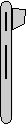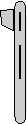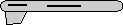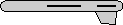# Maths - Quaternion to Axis-Angle - Sample Orientations

## Sample Rotations

In order to try to explain things and give some examples we can try I thought it might help to show the rotations for a finite subset of the rotation group. We will use the set of rotations of a cube onto itself, this is a permutation group which gives 24 possible rotations as explaned on this page.

In the following table we will need to know what quadrant the results are in, so I have taken some sample results from Math.atan2

heading applied first giving 4 possible orientations:reference orientation q = 1 sqrt(1-qw*qw) = 0 to avoid divide by zero just set axis to arbitary value (does not matter as angle is zero): angle =0 x = 1 y = 0 z = 0 angle = 0 degrees axis = 1,0,0rotate by 90 degrees about y axis q = 0.7071 + j 0.7071 sqrt(1-qw*qw) = 0.7071 angle = 2 * acos(qw) = 45*2=90degrees x = qx / sqrt(1-qw*qw) = y = qy / sqrt(1-qw*qw) = z = qz / sqrt(1-qw*qw) = angle = 90 degrees axis = 0,1,0rotate by 180 degrees about y axis q = j sqrt(1-qw*qw) = 1 angle = 2 * acos(qw) = 2*90=180deg x = qx / sqrt(1-qw*qw) = y = qy / sqrt(1-qw*qw) = z = qz / sqrt(1-qw*qw) = angle = 180 degrees axis = 0,1,0rotate by 270 degrees about y axis   q = 0.7071 - j 0.7071 (equivilant rotation to: -0.7071 + j 0.7071) sqrt(1-qw*qw) = 0.7071 angle = 2 * acos(qw) = 45*2=90degrees x = qx / sqrt(1-qw*qw) = y = qy / sqrt(1-qw*qw) = z = qz / sqrt(1-qw*qw) = angle = 90 degrees axis = 0,-1,0 or angle = -90 degrees axis = 0,1,0

Then apply attitude +90 degrees for each of the above: (note: that if we went on to apply bank to these it would just rotate between these values, the straight up and streight down orientations are known as singularities because they can be fully defined without using the bank value)q = 0.7071 + k 0.7071 sqrt(1-qw*qw) = 0.7071 angle = 2 * acos(qw) = 45*2=90degrees x = qx / sqrt(1-qw*qw) = y = qy / sqrt(1-qw*qw) = z = qz / sqrt(1-qw*qw) = angle = 90 degrees axis = 0,0,1q = 0.5 + i 0.5 + j 0.5 + k 0.5 sqrt(1-qw*qw) = 0.866 angle = 2 * acos(qw) = 2*60=120deg x = qx / sqrt(1-qw*qw) = y = qy / sqrt(1-qw*qw) = z = qz / sqrt(1-qw*qw) = angle = 120 degrees axis = 0.5774,0.5774,0.5774q = i 0.7071 +j 0.7071 sqrt(1-qw*qw) = 1 angle = 2 * acos(qw) = 2*90=180deg x = qx / sqrt(1-qw*qw) = y = qy / sqrt(1-qw*qw) = z = qz / sqrt(1-qw*qw) = angle = 180 degrees axis = 0.7071,0.7071,0q = 0.5 - i 0.5 - j 0.5 + k 0.5 sqrt(1-qw*qw) = 0.866 angle = 2 * acos(qw) = 2*60=120deg x = qx / sqrt(1-qw*qw) = y = qy / sqrt(1-qw*qw) = z = qz / sqrt(1-qw*qw) = angle = 120 degrees axis = -0.5774,-0.5774,0.5774

Or instead apply attitude -90 degrees (also a singularity):q = 0.7071 - k 0.7071 (equivilant rotation to: -0.7071 + k 0.7071) sqrt(1-qw*qw) = 0.7071 angle = 2 * acos(qw) = 45*2=90degrees x = qx / sqrt(1-qw*qw) = y = qy / sqrt(1-qw*qw) = z = qz / sqrt(1-qw*qw) = angle = 90 degrees axis = 0,0,-1 (equivilant rotation to: angle = -90 degrees axis = 0,0,1)q = 0.5 - i 0.5 + j 0.5 - k 0.5 sqrt(1-qw*qw) = 0.866 angle = 2 * acos(qw) = 2*60=120deg x = qx / sqrt(1-qw*qw) = y = qy / sqrt(1-qw*qw) = z = qz / sqrt(1-qw*qw) = angle = 120 degrees axis = -0.5774,0.5774,-0.5774q = -i 0.7071 + j 0.7071 (equivilant rotation to: i 0.7071 - j 0.7071) sqrt(1-qw*qw) = 1 angle = 2 * acos(qw) = 2*90=180deg x = qx / sqrt(1-qw*qw) = y = qy / sqrt(1-qw*qw) = z = qz / sqrt(1-qw*qw) = angle = 180 degrees axis = -0.7071,0.7071,0q = 0.5 + i 0.5 - j 0.5 - k 0.5 sqrt(1-qw*qw) = 0.866 angle = 2 * acos(qw) = 2*60=120deg x = qx / sqrt(1-qw*qw) = y = qy / sqrt(1-qw*qw) = z = qz / sqrt(1-qw*qw) =   angle = 120 degrees axis = 0.5774,-0.5774,-0.5774

Normally we dont go beond attitude + or - 90 degrees because thes are singularities, instead apply bank +90 degrees:q = 0.7071 + i 0.7071 sqrt(1-qw*qw) = 0.7071 angle = 2 * acos(qw) = 45*2=90degrees x = qx / sqrt(1-qw*qw) = y = qy / sqrt(1-qw*qw) = z = qz / sqrt(1-qw*qw) = angle = 90 degrees axis = 1,0,0q = 0.5 + i 0.5 + j 0.5 - k 0.5 sqrt(1-qw*qw) = 0.866 angle = 2 * acos(qw) = 2*60=120deg x = qx / sqrt(1-qw*qw) = y = qy / sqrt(1-qw*qw) = z = qz / sqrt(1-qw*qw) = angle = 120 degrees axis = 0.5774,0.5774,-0.5774q = j 0.7071 - k 0.7071 sqrt(1-qw*qw) = 1 angle = 2 * acos(qw) = 2*90=180deg x = qx / sqrt(1-qw*qw) = y = qy / sqrt(1-qw*qw) = z = qz / sqrt(1-qw*qw) = angle = 180 degrees axis = 0,0.7071,-0.7071q = 0.5 + i 0.5 - j 0.5 + k 0.5 sqrt(1-qw*qw) = 0.866 angle = 2 * acos(qw) = 2*60=120deg x = qx / sqrt(1-qw*qw) = y = qy / sqrt(1-qw*qw) = z = qz / sqrt(1-qw*qw) = angle = 120 degrees axis = 0.5774,-0.5774,0.5774

Apply bank +180 degrees:q = i sqrt(1-qw*qw) = 1 angle = 2 * acos(qw) = 2*90=180deg x = qx / sqrt(1-qw*qw) = y = qy / sqrt(1-qw*qw) = z = qz / sqrt(1-qw*qw) = angle = 180 degrees axis = 1,0,0q = i 0.7071 - k 0.7071 sqrt(1-qw*qw) = 1 angle = 2 * acos(qw) = 2*90=180deg x = qx / sqrt(1-qw*qw) = y = qy / sqrt(1-qw*qw) = z = qz / sqrt(1-qw*qw) = angle = 180 degrees axis = 0.7071,0,-0.7071q = k sqrt(1-qw*qw) = 1 angle = 2 * acos(qw) = 2*90=180deg x = qx / sqrt(1-qw*qw) = y = qy / sqrt(1-qw*qw) = z = qz / sqrt(1-qw*qw) = angle = 180 degrees axis = 0,0,1q = i 0.7071 + k 0.7071 sqrt(1-qw*qw) = 1 angle = 2 * acos(qw) = 2*90=180deg x = qx / sqrt(1-qw*qw) = y = qy / sqrt(1-qw*qw) = z = qz / sqrt(1-qw*qw) = angle = 180 degrees axis = 0.7071,0,0.7071

Apply bank -90 degrees:q = 0.7071 - i 0.7071 (equivilant rotation to: -0.7071 + i 0.7071) sqrt(1-qw*qw) = 0.7071 angle = 2 * acos(qw) = 45*2=90degrees x = qx / sqrt(1-qw*qw) = y = qy / sqrt(1-qw*qw) = z = qz / sqrt(1-qw*qw) = angle = 90 degrees axis = -1,0,0 (equivilant rotation to: angle = -90 degrees axis = 1,0,0)q = 0.5 - i 0.5 + j 0.5 + k 0.5 sqrt(1-qw*qw) = 0.866 angle = 2 * acos(qw) = 2*60=120deg x = qx / sqrt(1-qw*qw) = y = qy / sqrt(1-qw*qw) = z = qz / sqrt(1-qw*qw) = angle = 120 degrees axis = -0.5774,0.5774,0.5774q = j 0.7071 + k 0.7071 sqrt(1-qw*qw) = 1 angle = 2 * acos(qw) = 2*90=180deg x = qx / sqrt(1-qw*qw) = y = qy / sqrt(1-qw*qw) = z = qz / sqrt(1-qw*qw) = angle = 180 degrees axis = 0,0.7071,0.7071q = 0.5 - i 0.5 - j 0.5 - k 0.5 sqrt(1-qw*qw) = 0.866 angle = 2 * acos(qw) = 2*60=120deg x = qx / sqrt(1-qw*qw) = y = qy / sqrt(1-qw*qw) = z = qz / sqrt(1-qw*qw) = angle = 120 degrees axis = -0.5774,-0.5774,-0.5774